# User biglm

Senior Information Security lead at Bank of Israel
May. 1, 2015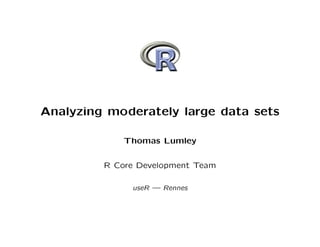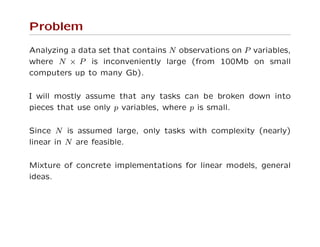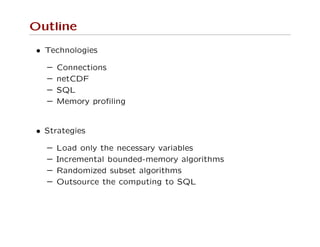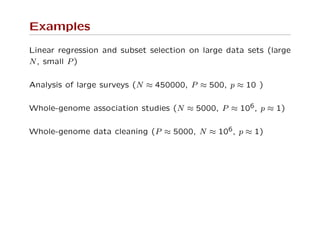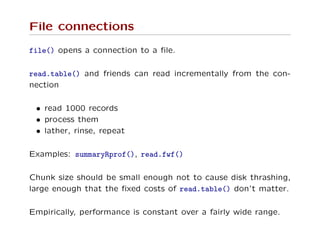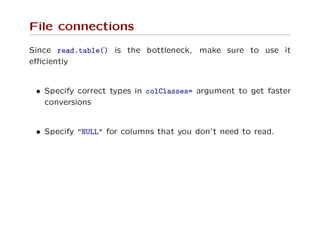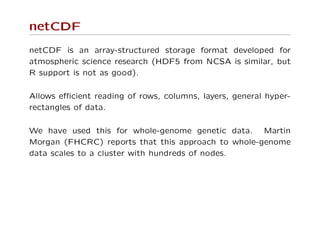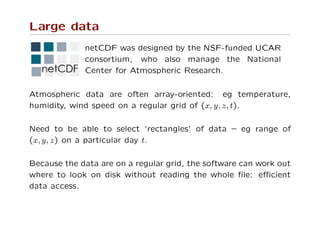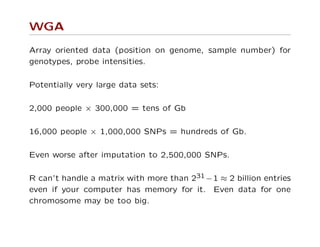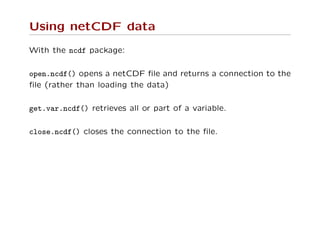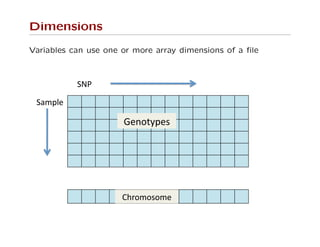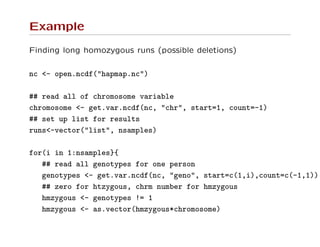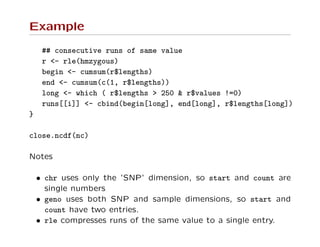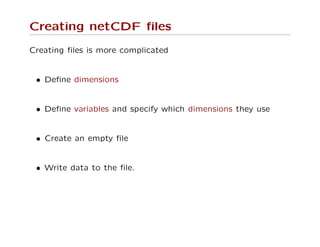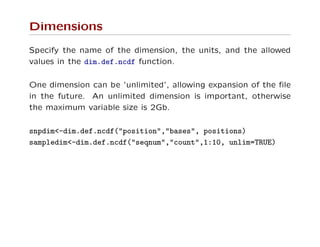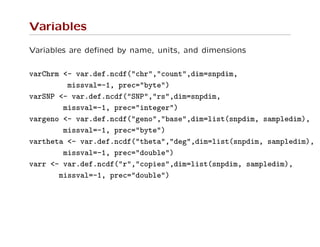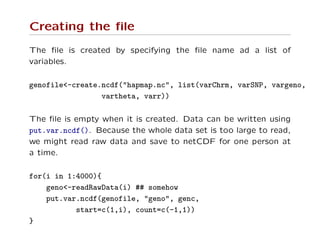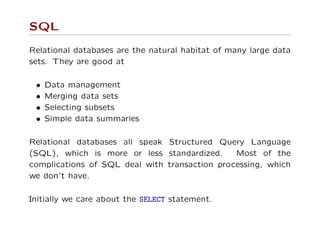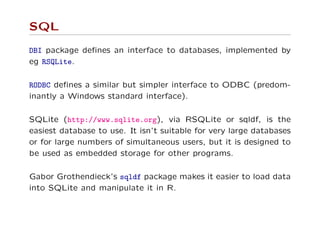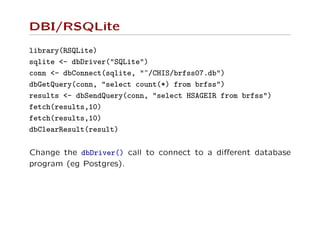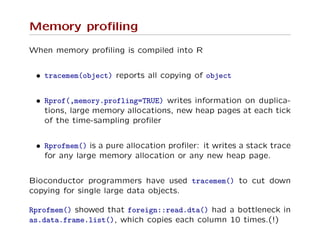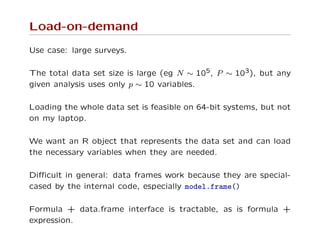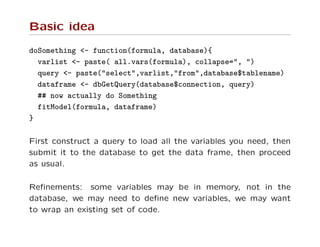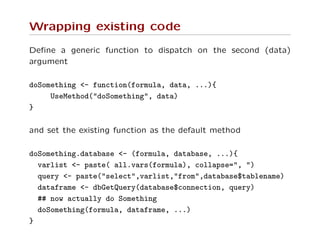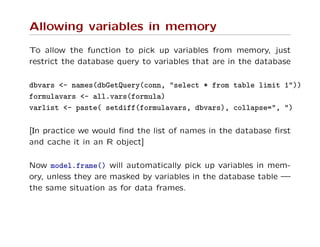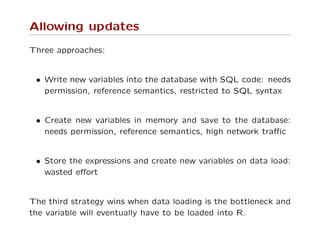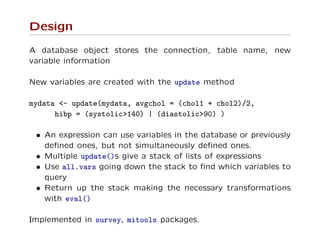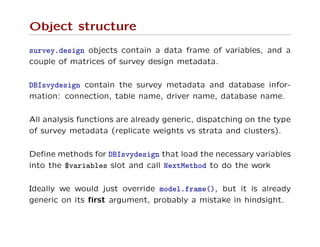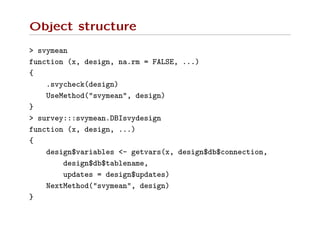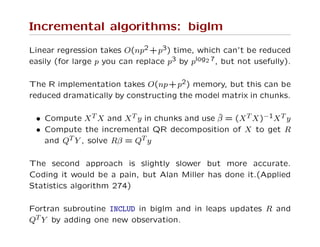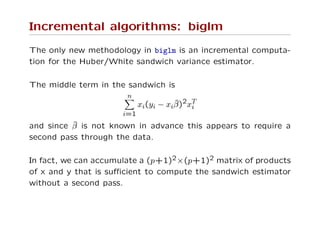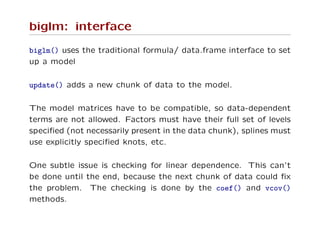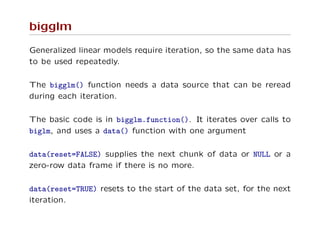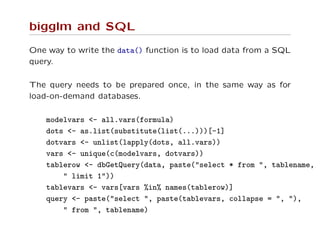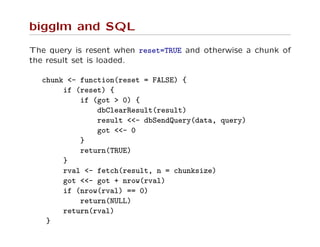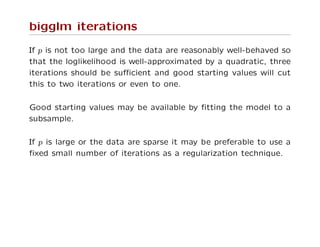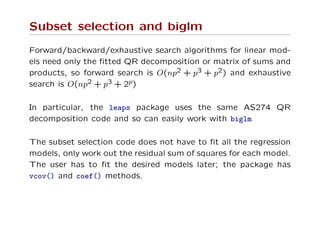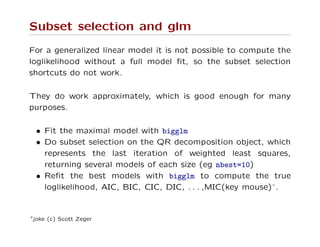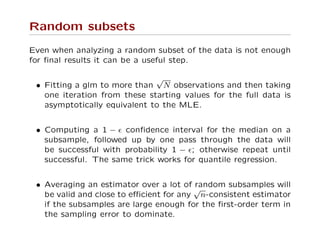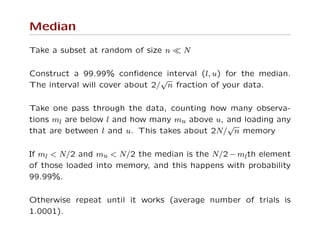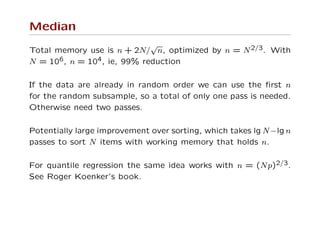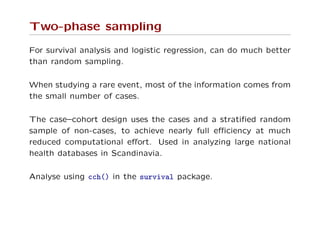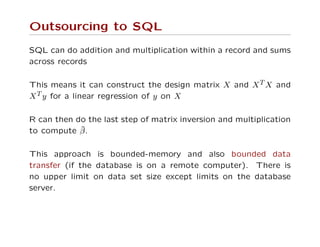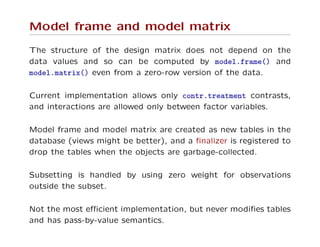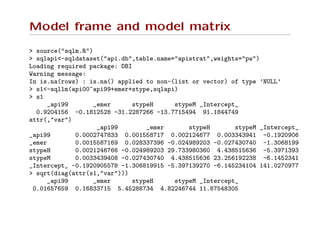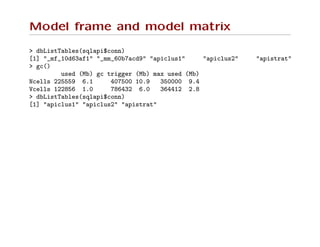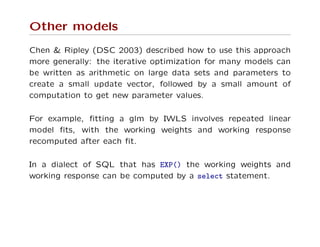1 of 48

### User biglm

• 1. Analyzing moderately large data sets Thomas Lumley R Core Development Team useR — Rennes
• 2. Problem Analyzing a data set that contains N observations on P variables, where N × P is inconveniently large (from 100Mb on small computers up to many Gb). I will mostly assume that any tasks can be broken down into pieces that use only p variables, where p is small. Since N is assumed large, only tasks with complexity (nearly) linear in N are feasible. Mixture of concrete implementations for linear models, general ideas.
• 3. Outline • Technologies – Connections – netCDF – SQL – Memory proﬁling • Strategies – Load only the necessary variables – Incremental bounded-memory algorithms – Randomized subset algorithms – Outsource the computing to SQL
• 4. Examples Linear regression and subset selection on large data sets (large N, small P) Analysis of large surveys (N ≈ 450000, P ≈ 500, p ≈ 10 ) Whole-genome association studies (N ≈ 5000, P ≈ 106, p ≈ 1) Whole-genome data cleaning (P ≈ 5000, N ≈ 106, p ≈ 1)
• 5. File connections file() opens a connection to a ﬁle. read.table() and friends can read incrementally from the con- nection • read 1000 records • process them • lather, rinse, repeat Examples: summaryRprof(), read.fwf() Chunk size should be small enough not to cause disk thrashing, large enough that the ﬁxed costs of read.table() don’t matter. Empirically, performance is constant over a fairly wide range.
• 6. File connections Since read.table() is the bottleneck, make sure to use it eﬃciently • Specify correct types in colClasses= argument to get faster conversions • Specify "NULL" for columns that you don’t need to read.
• 7. netCDF netCDF is an array-structured storage format developed for atmospheric science research (HDF5 from NCSA is similar, but R support is not as good). Allows eﬃcient reading of rows, columns, layers, general hyper- rectangles of data. We have used this for whole-genome genetic data. Martin Morgan (FHCRC) reports that this approach to whole-genome data scales to a cluster with hundreds of nodes.
• 8. Large data netCDF was designed by the NSF-funded UCAR consortium, who also manage the National Center for Atmospheric Research. Atmospheric data are often array-oriented: eg temperature, humidity, wind speed on a regular grid of (x, y, z, t). Need to be able to select ‘rectangles’ of data – eg range of (x, y, z) on a particular day t. Because the data are on a regular grid, the software can work out where to look on disk without reading the whole ﬁle: eﬃcient data access.
• 9. WGA Array oriented data (position on genome, sample number) for genotypes, probe intensities. Potentially very large data sets: 2,000 people × 300,000 = tens of Gb 16,000 people × 1,000,000 SNPs = hundreds of Gb. Even worse after imputation to 2,500,000 SNPs. R can’t handle a matrix with more than 231 −1 ≈ 2 billion entries even if your computer has memory for it. Even data for one chromosome may be too big.
• 10. Using netCDF data With the ncdf package: open.ncdf() opens a netCDF ﬁle and returns a connection to the ﬁle (rather than loading the data) get.var.ncdf() retrieves all or part of a variable. close.ncdf() closes the connection to the ﬁle.
• 11. Dimensions Variables can use one or more array dimensions of a ﬁle !"#\$ !%&'()\$ *)+,-.')/\$ 012,&,/,&)\$
• 12. Example Finding long homozygous runs (possible deletions) nc <- open.ncdf("hapmap.nc") ## read all of chromosome variable chromosome <- get.var.ncdf(nc, "chr", start=1, count=-1) ## set up list for results runs<-vector("list", nsamples) for(i in 1:nsamples}{ ## read all genotypes for one person genotypes <- get.var.ncdf(nc, "geno", start=c(1,i),count=c(-1,1)) ## zero for htzygous, chrm number for hmzygous hmzygous <- genotypes != 1 hmzygous <- as.vector(hmzygous*chromosome)
• 13. Example ## consecutive runs of same value r <- rle(hmzygous) begin <- cumsum(r\$lengths) end <- cumsum(c(1, r\$lengths)) long <- which ( r\$lengths > 250 & r\$values !=0) runs[[i]] <- cbind(begin[long], end[long], r\$lengths[long]) } close.ncdf(nc) Notes • chr uses only the ’SNP’ dimension, so start and count are single numbers • geno uses both SNP and sample dimensions, so start and count have two entries. • rle compresses runs of the same value to a single entry.
• 14. Creating netCDF ﬁles Creating ﬁles is more complicated • Deﬁne dimensions • Deﬁne variables and specify which dimensions they use • Create an empty ﬁle • Write data to the ﬁle.
• 15. Dimensions Specify the name of the dimension, the units, and the allowed values in the dim.def.ncdf function. One dimension can be ’unlimited’, allowing expansion of the ﬁle in the future. An unlimited dimension is important, otherwise the maximum variable size is 2Gb. snpdim<-dim.def.ncdf("position","bases", positions) sampledim<-dim.def.ncdf("seqnum","count",1:10, unlim=TRUE)
• 16. Variables Variables are deﬁned by name, units, and dimensions varChrm <- var.def.ncdf("chr","count",dim=snpdim, missval=-1, prec="byte") varSNP <- var.def.ncdf("SNP","rs",dim=snpdim, missval=-1, prec="integer") vargeno <- var.def.ncdf("geno","base",dim=list(snpdim, sampledim), missval=-1, prec="byte") vartheta <- var.def.ncdf("theta","deg",dim=list(snpdim, sampledim), missval=-1, prec="double") varr <- var.def.ncdf("r","copies",dim=list(snpdim, sampledim), missval=-1, prec="double")
• 17. Creating the ﬁle The ﬁle is created by specifying the ﬁle name ad a list of variables. genofile<-create.ncdf("hapmap.nc", list(varChrm, varSNP, vargeno, vartheta, varr)) The ﬁle is empty when it is created. Data can be written using put.var.ncdf(). Because the whole data set is too large to read, we might read raw data and save to netCDF for one person at a time. for(i in 1:4000){ geno<-readRawData(i) ## somehow put.var.ncdf(genofile, "geno", genc, start=c(1,i), count=c(-1,1)) }
• 18. SQL Relational databases are the natural habitat of many large data sets. They are good at • Data management • Merging data sets • Selecting subsets • Simple data summaries Relational databases all speak Structured Query Language (SQL), which is more or less standardized. Most of the complications of SQL deal with transaction processing, which we don’t have. Initially we care about the SELECT statement.
• 19. SQL SELECT var1, var2 FROM table WHERE condition SELECT sum(var1) as svar1, sum(var2) as svar2 FROM table GROUP BY category SELECT * FROM table limit 1 SELECT count(*) FROM table SELECT count(*) FROM table GROUP BY category
• 20. SQL DBI package deﬁnes an interface to databases, implemented by eg RSQLite. RODBC deﬁnes a similar but simpler interface to ODBC (predom- inantly a Windows standard interface). SQLite (http://www.sqlite.org), via RSQLite or sqldf, is the easiest database to use. It isn’t suitable for very large databases or for large numbers of simultaneous users, but it is designed to be used as embedded storage for other programs. Gabor Grothendieck’s sqldf package makes it easier to load data into SQLite and manipulate it in R.
• 21. DBI/RSQLite library(RSQLite) sqlite <- dbDriver("SQLite") conn <- dbConnect(sqlite, "~/CHIS/brfss07.db") dbGetQuery(conn, "select count(*) from brfss") results <- dbSendQuery(conn, "select HSAGEIR from brfss") fetch(results,10) fetch(results,10) dbClearResult(result) Change the dbDriver() call to connect to a diﬀerent database program (eg Postgres).
• 22. Memory proﬁling When memory proﬁling is compiled into R • tracemem(object) reports all copying of object • Rprof(,memory.profling=TRUE) writes information on duplica- tions, large memory allocations, new heap pages at each tick of the time-sampling proﬁler • Rprofmem() is a pure allocation proﬁler: it writes a stack trace for any large memory allocation or any new heap page. Bioconductor programmers have used tracemem() to cut down copying for single large data objects. Rprofmem() showed that foreign::read.dta() had a bottleneck in as.data.frame.list(), which copies each column 10 times.(!)
• 23. Load-on-demand Use case: large surveys. The total data set size is large (eg N ∼ 105, P ∼ 103), but any given analysis uses only p ∼ 10 variables. Loading the whole data set is feasible on 64-bit systems, but not on my laptop. We want an R object that represents the data set and can load the necessary variables when they are needed. Diﬃcult in general: data frames work because they are special- cased by the internal code, especially model.frame() Formula + data.frame interface is tractable, as is formula + expression.
• 24. Basic idea doSomething <- function(formula, database){ varlist <- paste( all.vars(formula), collapse=", ") query <- paste("select",varlist,"from",database\$tablename) dataframe <- dbGetQuery(database\$connection, query) ## now actually do Something fitModel(formula, dataframe) } First construct a query to load all the variables you need, then submit it to the database to get the data frame, then proceed as usual. Reﬁnements: some variables may be in memory, not in the database, we may need to deﬁne new variables, we may want to wrap an existing set of code.
• 25. Wrapping existing code Deﬁne a generic function to dispatch on the second (data) argument doSomething <- function(formula, data, ...){ UseMethod("doSomething", data) } and set the existing function as the default method doSomething.database <- (formula, database, ...){ varlist <- paste( all.vars(formula), collapse=", ") query <- paste("select",varlist,"from",database\$tablename) dataframe <- dbGetQuery(database\$connection, query) ## now actually do Something doSomething(formula, dataframe, ...) }
• 26. Allowing variables in memory To allow the function to pick up variables from memory, just restrict the database query to variables that are in the database dbvars <- names(dbGetQuery(conn, "select * from table limit 1")) formulavars <- all.vars(formula) varlist <- paste( setdiff(formulavars, dbvars), collapse=", ") [In practice we would ﬁnd the list of names in the database ﬁrst and cache it in an R object] Now model.frame() will automatically pick up variables in mem- ory, unless they are masked by variables in the database table — the same situation as for data frames.
• 27. Allowing updates Three approaches: • Write new variables into the database with SQL code: needs permission, reference semantics, restricted to SQL syntax • Create new variables in memory and save to the database: needs permission, reference semantics, high network traﬃc • Store the expressions and create new variables on data load: wasted eﬀort The third strategy wins when data loading is the bottleneck and the variable will eventually have to be loaded into R.
• 28. Design A database object stores the connection, table name, new variable information New variables are created with the update method mydata <- update(mydata, avgchol = (chol1 + chol2)/2, hibp = (systolic>140) | (diastolic>90) ) • An expression can use variables in the database or previously deﬁned ones, but not simultaneously deﬁned ones. • Multiple update()s give a stack of lists of expressions • Use all.vars going down the stack to ﬁnd which variables to query • Return up the stack making the necessary transformations with eval() Implemented in survey, mitools packages.
• 29. Object structure survey.design objects contain a data frame of variables, and a couple of matrices of survey design metadata. DBIsvydesign contain the survey metadata and database infor- mation: connection, table name, driver name, database name. All analysis functions are already generic, dispatching on the type of survey metadata (replicate weights vs strata and clusters). Deﬁne methods for DBIsvydesign that load the necessary variables into the \$variables slot and call NextMethod to do the work Ideally we would just override model.frame(), but it is already generic on its ﬁrst argument, probably a mistake in hindsight.
• 30. Object structure > svymean function (x, design, na.rm = FALSE, ...) { .svycheck(design) UseMethod("svymean", design) } > survey:::svymean.DBIsvydesign function (x, design, ...) { design\$variables <- getvars(x, design\$db\$connection, design\$db\$tablename, updates = design\$updates) NextMethod("svymean", design) }
• 31. Incremental algorithms: biglm Linear regression takes O(np2 +p3) time, which can’t be reduced easily (for large p you can replace p3 by plog2 7, but not usefully). The R implementation takes O(np+p2) memory, but this can be reduced dramatically by constructing the model matrix in chunks. • Compute XT X and XT y in chunks and use ˆβ = (XT X)−1XT y • Compute the incremental QR decomposition of X to get R and QT Y , solve Rβ = QT y The second approach is slightly slower but more accurate. Coding it would be a pain, but Alan Miller has done it.(Applied Statistics algorithm 274) Fortran subroutine INCLUD in biglm and in leaps updates R and QT Y by adding one new observation.
• 32. Incremental algorithms: biglm The only new methodology in biglm is an incremental computa- tion for the Huber/White sandwich variance estimator. The middle term in the sandwich is n i=1 xi(yi − xiˆβ)2xT i and since ˆβ is not known in advance this appears to require a second pass through the data. In fact, we can accumulate a (p+1)2×(p+1)2 matrix of products of x and y that is suﬃcient to compute the sandwich estimator without a second pass.
• 33. biglm: interface biglm() uses the traditional formula/ data.frame interface to set up a model update() adds a new chunk of data to the model. The model matrices have to be compatible, so data-dependent terms are not allowed. Factors must have their full set of levels speciﬁed (not necessarily present in the data chunk), splines must use explicitly speciﬁed knots, etc. One subtle issue is checking for linear dependence. This can’t be done until the end, because the next chunk of data could ﬁx the problem. The checking is done by the coef() and vcov() methods.
• 34. bigglm Generalized linear models require iteration, so the same data has to be used repeatedly. The bigglm() function needs a data source that can be reread during each iteration. The basic code is in bigglm.function(). It iterates over calls to biglm, and uses a data() function with one argument data(reset=FALSE) supplies the next chunk of data or NULL or a zero-row data frame if there is no more. data(reset=TRUE) resets to the start of the data set, for the next iteration.
• 35. bigglm and SQL One way to write the data() function is to load data from a SQL query. The query needs to be prepared once, in the same way as for load-on-demand databases. modelvars <- all.vars(formula) dots <- as.list(substitute(list(...)))[-1] dotvars <- unlist(lapply(dots, all.vars)) vars <- unique(c(modelvars, dotvars)) tablerow <- dbGetQuery(data, paste("select * from ", tablename, " limit 1")) tablevars <- vars[vars %in% names(tablerow)] query <- paste("select ", paste(tablevars, collapse = ", "), " from ", tablename)
• 36. bigglm and SQL The query is resent when reset=TRUE and otherwise a chunk of the result set is loaded. chunk <- function(reset = FALSE) { if (reset) { if (got > 0) { dbClearResult(result) result <<- dbSendQuery(data, query) got <<- 0 } return(TRUE) } rval <- fetch(result, n = chunksize) got <<- got + nrow(rval) if (nrow(rval) == 0) return(NULL) return(rval) }
• 37. bigglm iterations If p is not too large and the data are reasonably well-behaved so that the loglikelihood is well-approximated by a quadratic, three iterations should be suﬃcient and good starting values will cut this to two iterations or even to one. Good starting values may be available by ﬁtting the model to a subsample. If p is large or the data are sparse it may be preferable to use a ﬁxed small number of iterations as a regularization technique.
• 38. Subset selection and biglm Forward/backward/exhaustive search algorithms for linear mod- els need only the ﬁtted QR decomposition or matrix of sums and products, so forward search is O(np2 + p3 + p2) and exhaustive search is O(np2 + p3 + 2p) In particular, the leaps package uses the same AS274 QR decomposition code and so can easily work with biglm The subset selection code does not have to ﬁt all the regression models, only work out the residual sum of squares for each model. The user has to ﬁt the desired models later; the package has vcov() and coef() methods.
• 39. Subset selection and glm For a generalized linear model it is not possible to compute the loglikelihood without a full model ﬁt, so the subset selection shortcuts do not work. They do work approximately, which is good enough for many purposes. • Fit the maximal model with bigglm • Do subset selection on the QR decomposition object, which represents the last iteration of weighted least squares, returning several models of each size (eg nbest=10) • Reﬁt the best models with bigglm to compute the true loglikelihood, AIC, BIC, CIC, DIC, . . . ,MIC(key mouse)∗. ∗joke (c) Scott Zeger
• 40. Random subsets Even when analyzing a random subset of the data is not enough for ﬁnal results it can be a useful step. • Fitting a glm to more than √ N observations and then taking one iteration from these starting values for the full data is asymptotically equivalent to the MLE. • Computing a 1 − conﬁdence interval for the median on a subsample, followed up by one pass through the data will be successful with probability 1 − ; otherwise repeat until successful. The same trick works for quantile regression. • Averaging an estimator over a lot of random subsamples will be valid and close to eﬃcient for any √ n-consistent estimator if the subsamples are large enough for the ﬁrst-order term in the sampling error to dominate.
• 41. Median Take a subset at random of size n N Construct a 99.99% conﬁdence interval (l, u) for the median. The interval will cover about 2/ √ n fraction of your data. Take one pass through the data, counting how many observa- tions ml are below l and how many mu above u, and loading any that are between l and u. This takes about 2N/ √ n memory If ml < N/2 and mu < N/2 the median is the N/2 − mlth element of those loaded into memory, and this happens with probability 99.99%. Otherwise repeat until it works (average number of trials is 1.0001).
• 42. Median Total memory use is n + 2N/ √ n, optimized by n = N2/3. With N = 106, n = 104, ie, 99% reduction If the data are already in random order we can use the ﬁrst n for the random subsample, so a total of only one pass is needed. Otherwise need two passes. Potentially large improvement over sorting, which takes lg N−lg n passes to sort N items with working memory that holds n. For quantile regression the same idea works with n = (Np)2/3. See Roger Koenker’s book.
• 43. Two-phase sampling For survival analysis and logistic regression, can do much better than random sampling. When studying a rare event, most of the information comes from the small number of cases. The case–cohort design uses the cases and a stratiﬁed random sample of non-cases, to achieve nearly full eﬃciency at much reduced computational eﬀort. Used in analyzing large national health databases in Scandinavia. Analyse using cch() in the survival package.
• 44. Outsourcing to SQL SQL can do addition and multiplication within a record and sums across records This means it can construct the design matrix X and XT X and XT y for a linear regression of y on X R can then do the last step of matrix inversion and multiplication to compute ˆβ. This approach is bounded-memory and also bounded data transfer (if the database is on a remote computer). There is no upper limit on data set size except limits on the database server.
• 45. Model frame and model matrix The structure of the design matrix does not depend on the data values and so can be computed by model.frame() and model.matrix() even from a zero-row version of the data. Current implementation allows only contr.treatment contrasts, and interactions are allowed only between factor variables. Model frame and model matrix are created as new tables in the database (views might be better), and a ﬁnalizer is registered to drop the tables when the objects are garbage-collected. Subsetting is handled by using zero weight for observations outside the subset. Not the most eﬃcient implementation, but never modiﬁes tables and has pass-by-value semantics.
• 46. Model frame and model matrix > source("sqlm.R") > sqlapi<-sqldataset("api.db",table.name="apistrat",weights="pw") Loading required package: DBI Warning message: In is.na(rows) : is.na() applied to non-(list or vector) of type ’NULL’ > s1<-sqllm(api00~api99+emer+stype,sqlapi) > s1 _api99 _emer stypeH stypeM _Intercept_ 0.9204156 -0.1812528 -31.2287266 -13.7715494 91.1844749 attr(,"var") _api99 _emer stypeH stypeM _Intercept_ _api99 0.0002747833 0.001558717 0.002124677 0.003343941 -0.1920906 _emer 0.0015587169 0.028337396 -0.024989203 -0.027430740 -1.3068199 stypeH 0.0021246766 -0.024989203 29.733980360 4.438515636 -5.3971393 stypeM 0.0033439408 -0.027430740 4.438515636 23.256192238 -6.1452341 _Intercept_ -0.1920905578 -1.306819915 -5.397139270 -6.145234104 141.0270977 > sqrt(diag(attr(s1,"var"))) _api99 _emer stypeH stypeM _Intercept_ 0.01657659 0.16833715 5.45288734 4.82246744 11.87548305
• 47. Model frame and model matrix > dbListTables(sqlapi\$conn)  "_mf_10d63af1" "_mm_60b7acd9" "apiclus1" "apiclus2" "apistrat" > gc() used (Mb) gc trigger (Mb) max used (Mb) Ncells 225559 6.1 407500 10.9 350000 9.4 Vcells 122856 1.0 786432 6.0 364412 2.8 > dbListTables(sqlapi\$conn)  "apiclus1" "apiclus2" "apistrat"
• 48. Other models Chen & Ripley (DSC 2003) described how to use this approach more generally: the iterative optimization for many models can be written as arithmetic on large data sets and parameters to create a small update vector, followed by a small amount of computation to get new parameter values. For example, ﬁtting a glm by IWLS involves repeated linear model ﬁts, with the working weights and working response recomputed after each ﬁt. In a dialect of SQL that has EXP() the working weights and working response can be computed by a select statement.